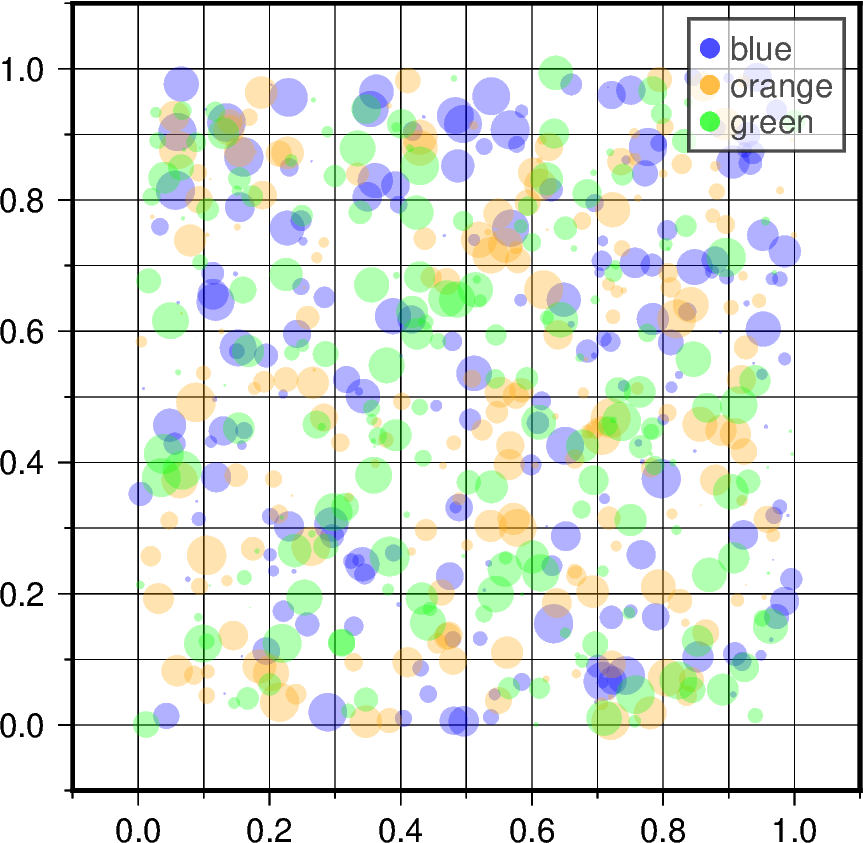# Scatter plots with a legend¶

To create a scatter plot with a legend one may use a loop and create one scatter plot per item to appear in the legend and set the label accordingly.

Modified from the matplotlib example: https://matplotlib.org/gallery/lines_bars_and_markers/scatter_with_legend.htmlOut:

<IPython.core.display.Image object>


import numpy as np
import pygmt

np.random.seed(19680801)
n = 200  # number of random data points

fig = pygmt.Figure()
fig.basemap(
region=[-0.1, 1.1, -0.1, 1.1],
projection="X10c/10c",
frame=["xa0.2fg", "ya0.2fg", "WSrt"],
)
for color in ["blue", "orange", "green"]:
x, y = np.random.rand(2, n)  # random X and Y data in [0,1]
sizes = np.random.rand(n) * 0.5  # random size [0,0.5], in cm
# plot data points as circles (style="c"), with different sizes
fig.plot(
x=x,
y=y,
style="c",
sizes=sizes,
color=color,
# Set the legend label,
# and set the circle size to be 0.25 cm (+S0.25c) in legend
label=f"{color}+S0.25c",
transparency=70,  # set transparency level for all symbols
)

fig.legend(transparency=30)  # set transparency level for legends
fig.show()


Total running time of the script: ( 0 minutes 0.751 seconds)

Gallery generated by Sphinx-Gallery